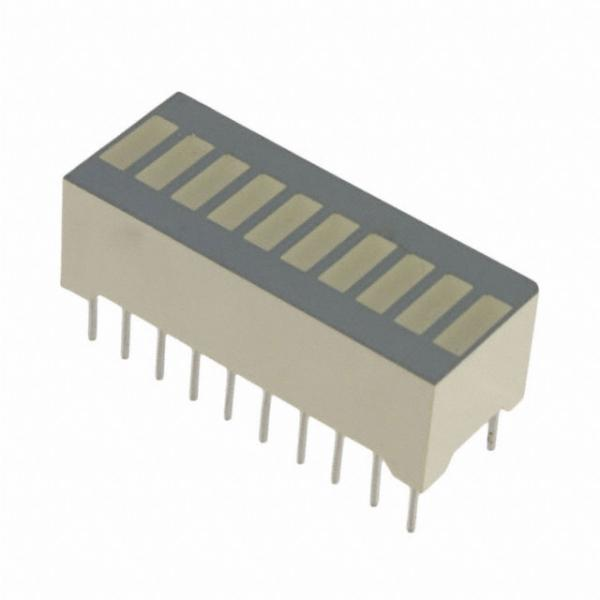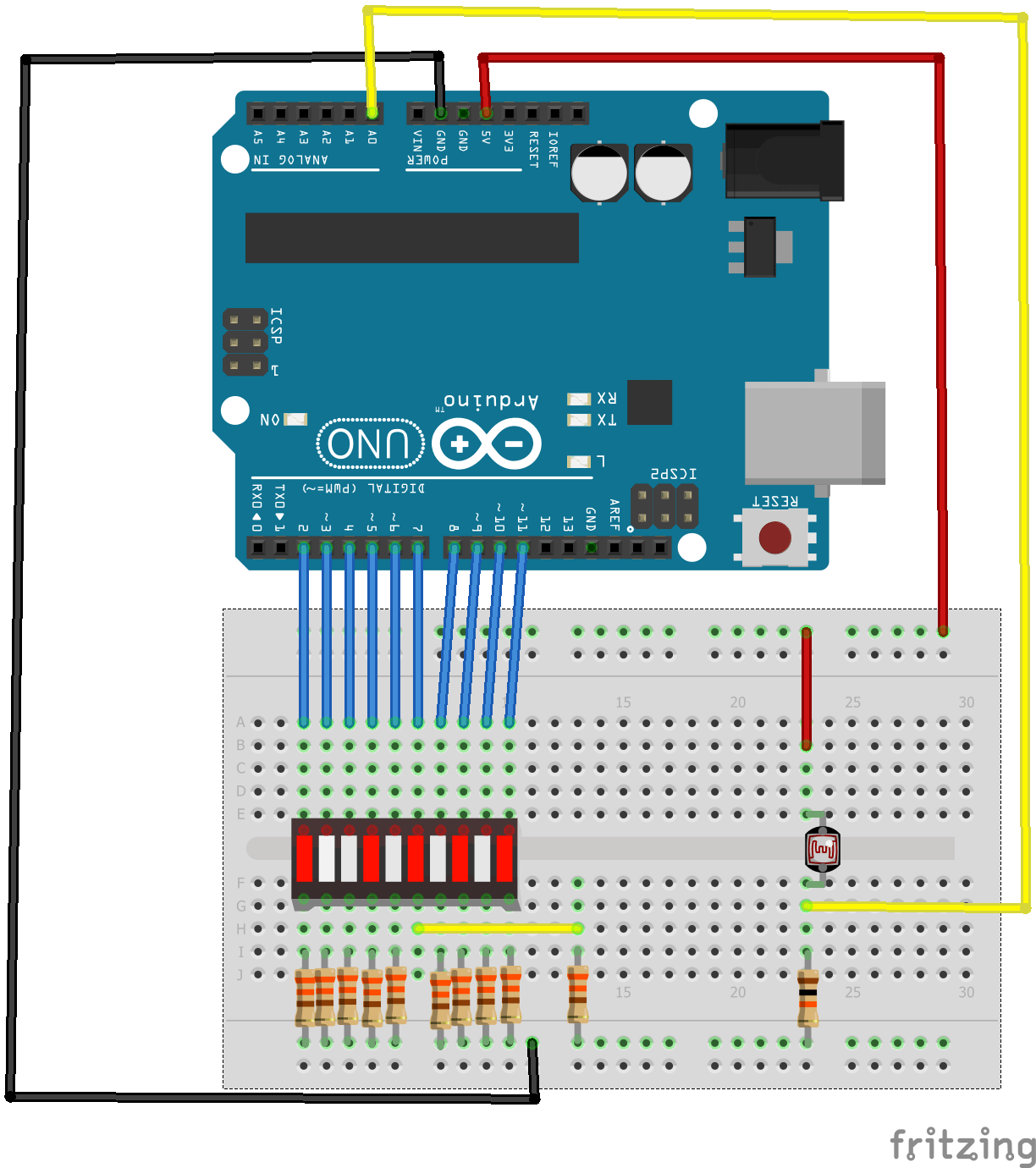Home Projects Arduino simple light meter

# Arduino simple light meter

In this example we will connect and LDR up to our Arduino and display the reading on a bargraph display, think of this as a low cost type of light meter.

A typical bargraph display looks like the one below, mine was labelled as a FJB10RParts List

You will need the following and also breadboard and cables.

Amount Part Type Properties
1 LED_BARGRAPH_10 segments 10; package led_bargaph_10
1 Arduino Uno (Rev3) type Arduino UNO (Rev3)
10 330Ω Resistor resistance 330Ω; tolerance ±5%; bands 4; package THT; pin spacing 400 mil
1 Photocell (LDR) resistance@ luminance 16 kOhms@ 10 lux; package THT; resistance@ dark 300 kOhms@ 10 seconds
1 10kΩ Resistor resistance 10kΩ; tolerance ±5%; bands 4; package THT; pin spacing 400 mil

Layout

Here is the layout, I connected the output from the LDR circuit to A0 and used D2 to D11 to connect to the bargraph, obviously this uses a lot of I/O so that is something you may want to think aboutCode

Very basic example here

[codesyntax lang=”cpp”]

```void setup ()
{
for (int i = 2; i <=11; i ++)
{
pinMode (i, OUTPUT);
}
}

void loop ()
{
int k = map (ldrvalue, 0, 1000, 2, 11);
for (int j = 3; j <14; j ++)
{
if (j <= k)
digitalWrite (j, HIGH);
else
digitalWrite (j, LOW);
}
}```

[/codesyntax]

Cover the LDR and shine light on it, you should varying amounts of LEDs on the bargraph lit